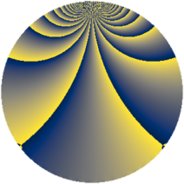# Properties

 Label 1045.2.bdLevel $1045$ Weight $2$ Character orbit 1045.bd Rep. character $\chi_{1045}(12,\cdot)$ Character field $\Q(\zeta_{12})$ Dimension $400$ Sturm bound $240$

# Related objects

## Defining parameters

 Level: $$N$$ $$=$$ $$1045 = 5 \cdot 11 \cdot 19$$ Weight: $$k$$ $$=$$ $$2$$ Character orbit: $$[\chi]$$ $$=$$ 1045.bd (of order $$12$$ and degree $$4$$) Character conductor: $$\operatorname{cond}(\chi)$$ $$=$$ $$95$$ Character field: $$\Q(\zeta_{12})$$ Sturm bound: $$240$$

## Dimensions

The following table gives the dimensions of various subspaces of $$M_{2}(1045, [\chi])$$.

Total New Old
Modular forms 496 400 96
Cusp forms 464 400 64
Eisenstein series 32 0 32

## Trace form

 $$400 q + 8 q^{6} + O(q^{10})$$ $$400 q + 8 q^{6} + 36 q^{15} + 176 q^{16} - 12 q^{17} - 48 q^{21} + 8 q^{23} - 12 q^{28} + 72 q^{32} - 60 q^{35} - 168 q^{36} - 68 q^{38} + 72 q^{40} - 88 q^{45} - 64 q^{47} - 84 q^{48} - 76 q^{57} + 96 q^{58} - 144 q^{60} - 80 q^{61} - 80 q^{63} + 96 q^{68} - 348 q^{72} + 64 q^{73} + 56 q^{76} - 120 q^{78} - 12 q^{80} + 216 q^{81} - 80 q^{82} - 8 q^{83} - 168 q^{86} + 208 q^{87} - 60 q^{90} + 4 q^{92} + 48 q^{93} - 8 q^{95} + 32 q^{96} + 36 q^{97} + 144 q^{98} + O(q^{100})$$

## Decomposition of $$S_{2}^{\mathrm{new}}(1045, [\chi])$$ into newform subspaces

The newforms in this space have not yet been added to the LMFDB.

## Decomposition of $$S_{2}^{\mathrm{old}}(1045, [\chi])$$ into lower level spaces

$$S_{2}^{\mathrm{old}}(1045, [\chi]) \cong$$ $$S_{2}^{\mathrm{new}}(95, [\chi])$$$$^{\oplus 2}$$